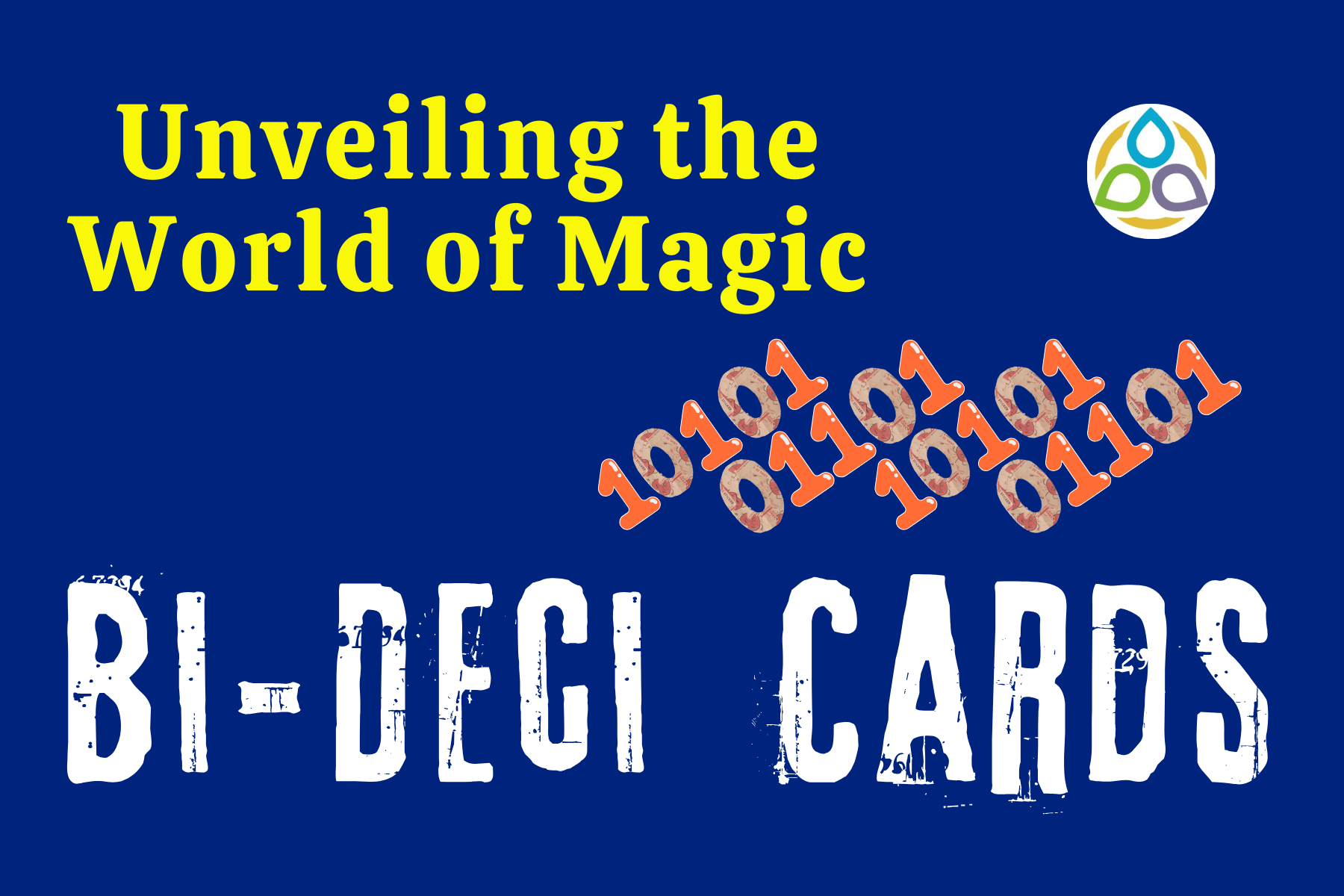## Unveiling the World of Magic Bi-Deci Cards

The mathematician does not study pure mathematics because it is useful; he studies it because he delights in it and he delights in it because it is beautiful.

Henri Poincare

A binary number is a number expressed in the base-2 numeral system, using only two symbols: 0 (zero) and 1 (one). The base-2 numeral system is a positional notation with a radix of 2, and each digit is referred to as a binary digit (or bit). The decimal (or base-10) number system, on the other hand, is a positional notation with a radix of 10, utilizing a set of 10 digits. Both the binary and decimal number systems can be employed to generate a stack of magical cards, as we’ll explore in this blog post.

The following five cards uniquely represent the numbers from 1 to 31, and no two numbers share the same appearance on the cards due to their distinct representations in base two (details are provided later). Let’s delve into playing with these cards.

Secretly choose any number from 1 to 31. Using the cards labeled A, B, C, D, and E, identify on which card(s) that particular number appears. Once you share this information, I can reveal your chosen number—like magic! Interested? Let’s consider an example.

Suppose you’ve secretly chosen a number, and you observe its presence on cards A, B, C, and D. Based on this information, I can deduce that your chosen number is 15. Want to try it again? No problem, let’s perform the magic once more. This time, secretly choose a number and identify its presence on various cards. Let’s say you see the number on cards A, B, C, D, and E. I’m confident your chosen number was 31! Intrigued about how I can guess it?

In the title of this game, bi and deci are respectively the short forms of binary and decimal. We can play the game in both ways—using binary and decimal formats, hence the name. Since the binary form of decimal numbers is crucial, let’s recall the concept of converting a number to its binary form, focusing on numbers from 1 to 31. If you’re not familiar with the corresponding binary forms, the following table might be helpful:

In this table, each decimal number corresponds to a five-bit binary representation. The rightmost bit corresponds to card A, followed by cards B, C, D, and the leftmost bit corresponds to card E. Here, 1 represents the presence, and 0 represents the absence of the particular card. For example, if we assign 1 to A and 0 to B, the number is present in card A but absent in card B.

Consider the first example. If the number is present on cards A, B, C, and D, it’s obvious that the number is absent on card E. Therefore, EDCBA ≡ 01111, and from the chart, this number represents 15. In the second example, where the card is present on all five cards, EDCBA ≡ 11111, corresponding to the number 31. This is the binary way.

There’s another way (the decimal way) to determine the desired number once the appearance of the number on specific cards is known. Consider the first instance from the examples above. Since the number is present on cards A, B, C, and D, simply add all the first numbers appearing on the cards: 1 + 2 + 4 + 8 = 15, as expected. For the other example, where the number is present on all five cards, add all the first numbers to get 1 + 2 + 4 + 8 + 16 = 31, as before.

This blog is as much yours as it is mine. Would you like to contribute an exceptional, non-routine article and have it published on Math1089? Perhaps you have a preliminary idea that you wish to see in its published form—please share your ideas by dropping us a line.

We wholeheartedly welcome your contributions and eagerly anticipate featuring your ideas on “Math1089 Mathematics for All” in our next captivating mathematics blog post. Thank you for being a part of our journey, and we look forward to your involvement in shaping the future content of Math1089. See you soon for another intriguing exploration into the world of mathematics!